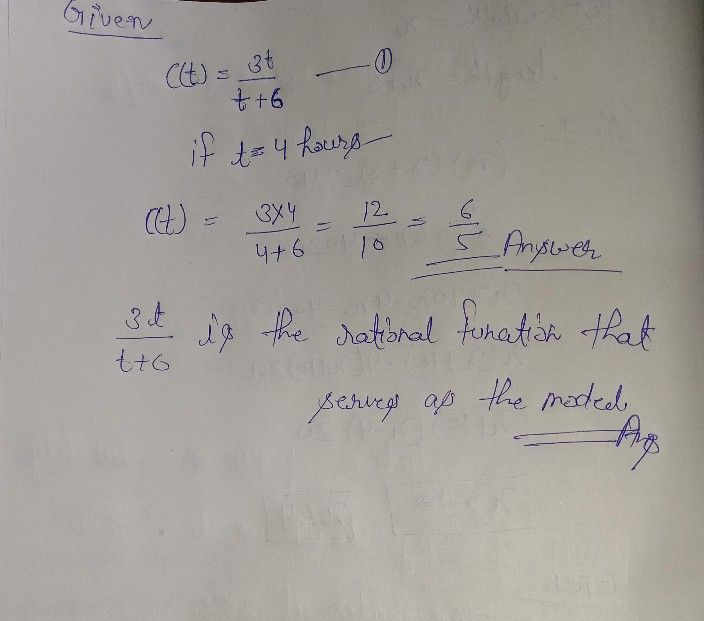Symbol
ProblemMedicine Dosage $LetC\left(t\right)=\dfrac {3t} {t+6}$ be the function that describes the concentration of a certain medication in the bloodstream overtime $t1f4$ hours have passed after the medicine was intake, how concentrated is it in the blood? $1c12$ a. What is the rational function that serves $0s$ the model? b. How are you going to determine the concentration of the medicine given the rational function and the number of hours?
10th-13th grade
Algebra
Search count: 105
SolutionQanda teacher - henrysee